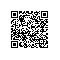# C/C++迭代器使用具体解释

vector<int>::iterator iter;

# begin 和 end 操作

vector<int>::iterator iter = ivec.begin();

【备注：不用操心begin和end在循环中的条件推断。大胆使用吧！

# vector 迭代器的自增和解引用运算

*iter = 0;

# 迭代器应用的程序演示样例

## 1、使用迭代器和下标改变vector的内容

#include <iostream>
#include <string>
#include <vector>

int print_int_vector(std::vector<int> ivec)
{
for(std::vector<int>::size_type ix =0, j = 0; ix != ivec.size(); ++ix, ++j)
{
std::cout<<ivec[ix]<<" "; //加空格！
}
std::cout<<std::endl;
return 0;
}

int main()
{
std::vector<int> ivec(10, 68); // empty vector
print_int_vector(ivec);
// reset all the elements in ivec to 0
/*
// 使用下标
for (std::vector<int>::size_type ix = 0; ix != ivec.size(); ++ix)
{
ivec[ix] = 0;
}
*/
// equivalent loop using iterators to reset all the elements in ivec to 0
for (std::vector<int>::iterator iter = ivec.begin(); iter != ivec.end(); ++iter)
*iter = 0; // set element to which iter refers to 0
print_int_vector(ivec);
return 0;
}

## 2、tuple功能的实现【不可变性】

const_iterator类型仅仅能用于读取容器内元素。但不能改变其值。

【注意：不要把 const_iterator 对象与 const 的 iterator 对象混淆起来。声明一个 const 迭代器时，必须初始化迭代器。一旦被初始化后。就不能改变它的值。】
vector<int> nums(10); // nums is nonconst
const vector<int>::iterator cit = nums.begin();
*cit = 1; // ok: cit can change its underlying element
++cit; // error: can't change the value of cit
【注意：const_iterator 对象能够用于 const vector 或非 const vector，由于不能改写元素值。

const 迭代器这样的类型差点儿没什么用处：一旦它被初始化后，仅仅能用它来改写其指向的元素，但不能使它指向不论什么其它元素。

tuple不可变的实现须要使用const声明。const vector<int> nums(10, 9);

## 总结

1、const_iterator须要注意：这个vector本身还是可变的，仅仅只是对const_iterator类型解引用的对象不可变。

2、const迭代器也就是仅仅能指向其所指向的元素。不能通过++等操作去指向其它元素。可是，所指向这个元素能够改变。

3、须要定义真正tuple，那就用const vector<int> nums(10, 9);来定义！

# 迭代器的算术操作

1、能够对迭代器对象加上或减去一个整形值。这样做将产生一个新的迭代器，其位置在 iter 所指元素之前（加）或之后（减） n 个元素的位置。

int main()
{
std::vector<int> ivec(10, 68);
print_int_vector(ivec);
int i = 0;
for (std::vector<int>::iterator iter = ivec.begin(); iter != ivec.end(); ++iter, i++)
*iter = i; // set element to which iter refers to i
print_int_vector(ivec);
std::vector<int>::iterator iter = ivec.begin();
iter += 100;
std::cout<<*iter;

return 0;
}

2、iter1 - iter2：

iter1 与 iter2 两者必须都指向同一 vector 中的元素，或者指向 vector 末端之后的下一个元素。

3、能够用迭代器算术操作来移动迭代器直接指向某个元素，比如。以下语句直接定位于 vector 中间元素：
vector<int>::iterator mid = vi.begin() + vi.size() / 2;

4、不论什么改变 vector 长度的操作都会使已存在的迭代器失效。

*iter = i; // set element to which iter refers to i
ivec.push_back(i*2);

{
*iter = i; // set element to which iter refers to i
ivec.push_back(i*2);
}使用钉钉扫一扫加入圈子
+ 订阅